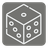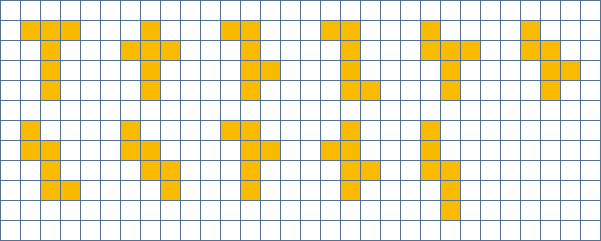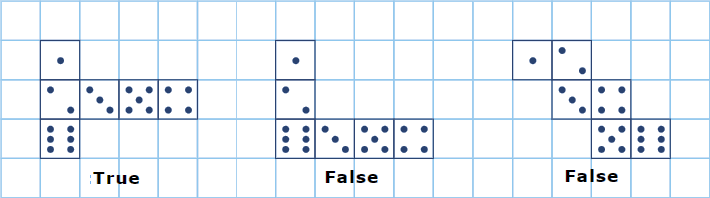Paper Dice
Simple+
English

This mission is the advanced version of the 'Identify Block' mission.

You have to write a code to check the development of drawing a dice.

The input represents the development of drawing one dice. It's a list of strings. These strings are of the same length. They consist of 1 to 6 digits and a space. These digits represent each side of the dice.

• If the development is correct, return True.
• If the followng cases, return False:
• You can't complete a cube.
• The sum of the top and bottom sides doesn't always equals 7.

11 nets of a cube.Example:

```paper_dice(['  1  ',
' 235 ',
'  6  ',
'  4  ']) == True

paper_dice(['    ',
'12  ',
' 36 ',
'  54',
'    ']) == True

paper_dice(['123456']) == False
paper_dice(['123  ',
'  456']) == False```

Input: A list of strings.

Output: The answer as a boolean.

Precondition:

• all(len(row) == len(paper) for row in paper)
• all((cell in '123456 ' for cell in row) for row in paper)